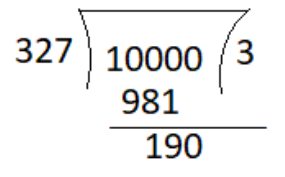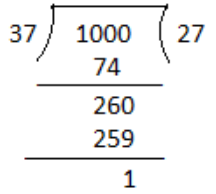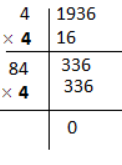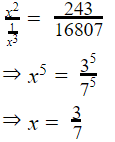# Number System Questions : SSC CPO 2021

Validity: 9 Months
What you will get
Course Highlights
• Based on latest Pattern
• English Medium eBooks
SSC

In this article, we are providing previous year's important questions and solutions of SSC CPO of number system asked by TCS. It is helpful for upcoming exams of SSC, CGL, CHSL, and other competitive exams conducted by TCS.

## Number System Important Questions

Q1. When the integer n is divided by 7, the remainder is 3. What is the remainder if 5n is divided by 7?

जब पूर्णांक n को 7 से विभाजित किया जाता है, तो शेषफल 3 आता है | जब 5n को 7 से विभाजित किया जाएगा तो शेषफल क्या आएगा ?

SSC CPO 16 March 2019 (Evening)

(a) 3

(b) 0

(c) 1

(d) 2

Q2. The number 23474 is exactly divisible by /संख्या 23474 विभाज्य है:

SSC CPO 14 March 2019 (Morning)

(a) 2 and 3 only

(b) 2 and 4 only

(c) 2 and 11 only

(d) 2 only

Q3. The least number that should be added to 10000 so that it is exactly divisible by 327 is:

10000 में न्यूनतम कौन सी संख्या जोड़ी जानी चाहिए ताकि यह 327 से पूर्णतः विभाजित हो जाए ?

SSC CPO 15 March 2019 (Morning)

(a) 327

(b) 237

(c) 137

(d) 190

Q4. Which least number should be added to 1000 so that the number obtained is exactly divisible by 37?

1000 में कम से कम कौन सी संख्या जोड़ी जानी चाहिए ताकि प्राप्त होने वाली संख्या 37 से पूर्णतः विभाजित हो ?

SSC CPO 16 March 2019 (Afternoon)

(a) 1

(b) 25

(c) 36

(d) 13

Q5. In number 16008, The numeral 6 has a face value:

16008 में अंक 6 का जातीय मान (face value) है :

SSC CPO 15 March 2019 (Evening)

(a) 6000

(b) 6

(c) 60

(d) 600

Q6. What is the difference between the largest and smallest numbers of the four digits created using numbers 2, 9, 6, 5? | (Each number can be used only once)

अंक 2,9,6, और 5 का उपयोग करके बनी चार अंको की सबसे बड़ी और सबसे छोटी संख्यायों का अंतर क्या है ? |(प्रत्येक अंक केवल एक ही बार प्रयुक्त हो सकता है )

SSC CPO 14 March 2019 (Evening)

(a) 6993

(b) 7056

(c) 6606

(d) 7083

Q7. A gardener planted 1936 saplings in a garden such that there were as many rows of saplings as the columns. The number of rows planted is:

एक माली ने किसी उद्यान में 1936 पौधे इस प्रकार लगाए कि पौधों की पंक्तियाँ तथा कतार बराबर थे |   पंक्तियों की संख्या ज्ञात करें |

SSC CPO 16 March 2019 (Afternoon)

(a) 46

(b) 44

(c) 48

(d) 42

Q8. The sum of all possible three-digit numbers formed by digits 3, 0, and 7, using each digit only once is:

अंक 3, 0 और 7 में से प्रत्येक अंक का केवल एक ही बार प्रयोग करके बनने वाली सभी संभावित तीन अंकों की संख्याओं का योग ज्ञात करें |

SSC CPO 14 March 2019 (Morning)

(a) 2010

(b) 1990

(c) 2220

(d) 2110

Q9. The square root of which of the following is a rational number

SSC CPO 12 March 2019 (Evening)

(a) 5823.82

(b) 22504.9

(c) 2460.14

(d) 1489.96

Q10. What is the sum of digits of the least number, which when divided by 15, 18 and 42 leaves the same remainder 8 in each case and is also divisible by 13?

SSC CPO 13 March 2019 (Evening)

(a) 25

(b) 24

(c) 22

(d) 26

Q11. The square root which of the following is a rational number?

SSC CPO 13 March 2019 (Evening)

(a) 5535.36

(b) 3152.88

(c) 72905.2

(d) 67508.5

Q12. To what power -3 should be raised to get -2187?

SSC CPO 14 March 2019 (Morning)

(a) 5

(b) 7

(c) -7

(d) -5

Q13.The cube root of 3375 is equal to:

SSC CPO 15 March 2019 (Morning)

(a) 35

(b) 25

(c) 55

(d) 15

Q14. To get a perfect square, what should the minimum number be added to 8212.

SSC CPO 15 March 2019 (Evening)

(a) 123

(b) 69

(c) 112

(d) 54

### Sol 1.

Ans. (c)

Let n be any number that gives remainder 3 when divided by 7.

For example 10.

5n = 50

Required remainder = 50/7= 1

### Sol 3. (c)Required number = 327-190 = 137

### Sol 4. (c)Least number to be needed = 37-1 = 36

### Sol 5.

Ans. (b)

Face value of 6 in 16008 is 6. As it is the value itself.

### Sol 6.

Ans. (d)

Difference between the largest and the smallest

9652-2569 = 7083

### Sol 7.

Ans. (b) 44### Sol 8.

Ans. (d)

Possible numbers are 307, 370, 703, and 730.

Therefore, Their Sum = 2110

Sol 9. (d) Going through the options:

Option (d) √1489.96=38.6

Hence required rational number is 1489.96

Sol 10. (d) LCM of (15, 18 and 42) = 630

Let the number be (630k+8).

Minimum value of k for which (630k+8) is divisible by 13, is equal to  3.

Hence the number (630k+8) = {630(3)+8} = 1898.

Sum of digits = 1+8+9+8 = 26

Sol 11. (a)

Going through the options:

Option (a) √5535.36=74.4

Hence required answer rational number is 5535.36.

Sol 12. (b) let the required power = n

According to the question,

(-3)n = -2187

= (-3)7

Therefore, Required power will be 7.

Sol 13. (d)

3375 =5 = 15

Sol 14. (b)  closest square to 8212 is 8281.

Therefore, 69 is to be added to 8212 to make it a perfect square.

Sol 15. (c)

Let the number = x## You may like similar articles on this website

You can call for any help/support/query  Mob: 9817390373, WhatsApp: 8295688244